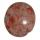Swimming pool 4

The pool shaped cuboid measuring 12.5 m × 640 cm at the bottom is 960hl water. To what height in meters reaches the water level?

Result

x =  1.2 m

Solution:Leave us a comment of example and its solution (i.e. if it is still somewhat unclear...):Be the first to comment!To solve this example are needed these knowledge from mathematics:

Do you know the volume and unit volume, and want to convert volume units?

Next similar examples:

1. Water poolWhat water level is in the pool shaped cuboid with bottom dimensions of 25 m and 10 meters, when is 3750hl water in the pool.
2. Oak cuboidOak timber is rectangular shaped with dimensions of 2m, 30 cm and 15 cm. It weight is 70 kg. Calculate the weight 1 dm³ of timber.
3. StoneWhen Peter threw stone in a box of water he discovered that the water level has risen by 9 cm. The box has a cuboid shape, the bottom has dimensions of 24 cm and 14 cm, height is 50 cm. What volume has a stone?
4. Fire tankHow deep is the fire tank with the dimensions of the bottom 7m and 12m, when filled with 420 m3 of water?
5. TankTo cuboid tank whose bottom has dimensions of 9 m and 15 m were flow 1080 hectoliters of water. This was filled 40% of the tank volume. Calculate the depth of the tank.
6. AquariumAquarium is rectangular box with square base containing 76 liters of water. Length of base edge is 42 cm. To what height the water level goes?
7. Reservoir 3How many cubic feet of water is stored in the reservoir that has a length of 200 feet, a width of 100 feet, an overflow depth of 32 feet, and a current water level of 24 feet?
8. Water lakeThe length of the lake water is 8 meters width 7 meters and depth 120 centimeters. How many liters of water can fit into the water lake?
9. Cuboid aquariumCuboid 25 times 30 cm. How long is third side if cuboid contains 30 liters of water?
10. Water reservoirThe water tank has a cuboid with edges a= 1 m, b=2 m , c = 1 m. Calculate how many centimeters of water level falls, if we fill fifteen 12 liters cans.
11. HectolitersHow many hectoliters of water fits into cuboid tank with dimensions of a = 3.5 m b = 2.5 m c = 1.4 m?
12. Glass doorWhat is the weight of glass door panel 5 mm thick height 2.1 meters and a width of 65 cm and 1 cubic dm of glass weighs 2.5 kg?
13. Concrete boxThe concrete box with walls thick 5 cm has the following external dimensions: length 1.4 m, width 38 cm and height 42 cm. How many liters of soil can fit if I fill it to the brim?
14. Two cuboidsFind the volume of cuboidal box whose one edge is: a) 1.4m and b) 2.1dm
15. Liters in cylinderDetermine the height at which level 24 liters of water in a cylindrical container having a bottom diameter 36 cm.
16. The shopThe shop has 3 hectoliters of water. How many liter bottles is it?
17. Cylindrical tank 2If a cylindrical tank with volume is used 12320cm raised to the power of 3 and base 28cm is used to store water. How many liters of water can it hold?